# How to Count unique entries in a range using COUNTIF function in Excel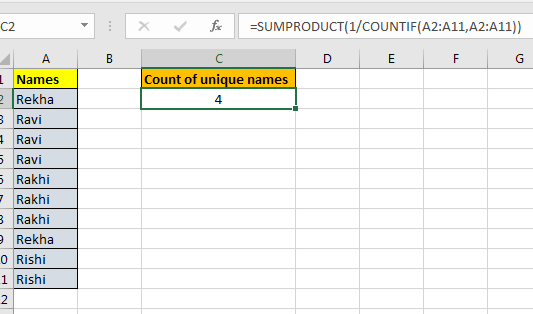In this article, we will learn How to Count unique entries in a range using COUNTIF function in Excel

.What are unique values in range ?

Non- repeating values are called unique values. For example : In a list of groceries Rice, Wheat, Floor and Wheat. Values Rice & Floor are unique or non repeating whereas as wheat is repeating and non unique values. Sometimes in excel in a long list we need to find the count of unique value

COUNTIF Formula to get unique value count in Excel

Here we will use the combination of SUMPRODUCT and COUNTIF function. Here COUNTIF function counts the number of values in range. SUMPRODUCT finds the sum of values for only the corresponding 1s.

Formula syntax:

 =SUMPRODUCT(1/COUNTIF(range,range))

range : list of values in given range

Example :

All of these might be confusing to understand. Let's understand how to use the function using an example. Here Let's say we have a name list. Now this list may contain duplicate values. How can we count unique names only? See below table.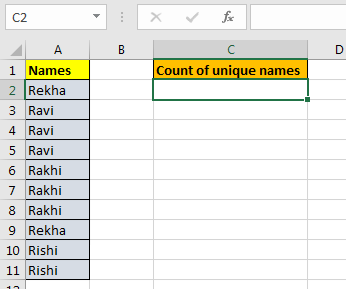Write this Unique Count formula anywhere on the sheet. And you will have a unique count of items in a given range.

 =SUMPRODUCT(1/COUNTIF(A2:A11,A2:A11))#### Explanation:

• COUNTIF(A2:A11,A2:A11) : This part will return an array of count of each element in cell. If you select this part of formula and press F9, you will see the array. If “radha” occurs two times and “Reena” 3 then array will be. {2,2,3,3,3}.In this example it is {2;3;3;3;3;3;3;2;2;2}.
• 1/COUNTIF(A2:A11,A2:A11) : Here we are dividing 1 by each element of the array returned by COUNTIF(A2:A11,A2:A11).  It will again return an array of fractions. If array returned by countif function is {2,2,3,3,3} then the fraction array will be {0.5,0.5,0.333,0.333,0.333}. If we some them then we will get {1,1}. 1 for each element in array. For our example it is {0.5;0.333;0.333;0.333;0.333;0.333;0.333;0.5;0.5;0.5}.

Still with me? OK, we are almost there.

• SUMPRODUCT(1/COUNTIF(A2:A11,A2:A11)) : It will sum the array provided by 1/COUNTIF(A2:A11,A2:A11), which will be the count of unique values in range.

Extract unique or non recurring values using UNIQUE function in Excel 365 or spreadsheet

Extract unique numbers from the list using UNIQUE function of Excel 365.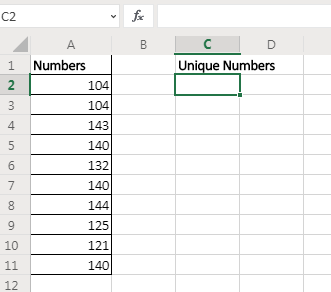Here I have sample data. In range A2:A11 I have some numbers that contain duplicate values. I want to get unique values from this range. I will simply use the UNIQUE function of Excel 365.

 =UNIQUE(A2:A11)

This formula simply returns all unique values from the range.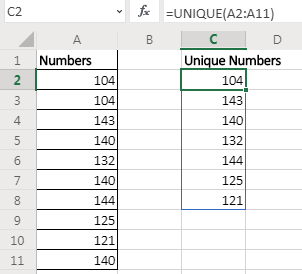As soon as you hit the enter button, the resultant array of unique value is spilled down the cells. This is a dynamic array that is only available for Excel 365 online.

Example 2: Extract Values that only occur once in the range.

In the above example, we got unique values from the range. If a value was occurring once, twice, or more we got only one stance of it. But if we want to extract values that only occurs once in the range (unique values in range) then the formula will be:

 =UNIQUE(A2:A11,1)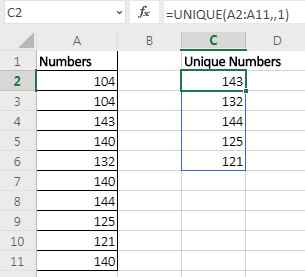Here we set the exactly_once variable to TRUE. This returns the value that are unique in the range/array itself.

Here are all the observational notes using the formula in Excel
Notes :

1. The formula works with numbers, text, date values, etc.
2. Use the same data type to match criteria in criteria list like name in names list, date in date values.
3. Lists as criteria ranges must be of same length, or the formula returns #VALUE! error.
4. Operators like equals to ( = ), less than equal to ( <= ), greater than ( > ) or not equals to ( <> ) can be used in criteria arguments, with numbers list only.
5. For example matching time values which have hour value 6. So you can use "06:*:*". Each ( * ) asterisk character is used for the uncertain minutes and seconds.

Hope this article about How to Count unique entries in a range using COUNTIF function in Excel is explanatory. Find more articles on counting values and related Excel formulas here. If you liked our blogs, share it with your friends on Facebook. And also you can follow us on Twitter and Facebook. We would love to hear from you, do let us know how we can improve, complement or innovate our work and make it better for you. Write to us at info@exceltip.com.

Related Articles :

How to use the COUNTIF Function in Excel : Count values with conditions using this amazing function. You don't need to filter your data to count specific values. Countif function is essential to prepare your dashboard.

How to use the SUMPRODUCT function in Excel: Returns the SUM after multiplication of values in multiple arrays in excel.

COUNTIFS with Dynamic Criteria Range : Count cells selecting the criteria from the list of options in criteria cell in Excel using data validation tool.

COUNTIFS Two Criteria Match : multiple criteria match in different lists in table using the COUNTIFS function in Excel

COUNTIFS With OR For Multiple Criteria : match two or more names in the same list using the OR criteria applied on the list in Excel.

How to Use Countif in VBA in Microsoft Excel : Count cells with criteria using Visual Basic for Applications code in Excel macros.

How to use wildcards in excel : Count cells matching phrases in text lists using the wildcards ( * , ? , ~ )in excel

Popular Articles:

How to use the IF Function in Excel : The IF statement in Excel checks the condition and returns a specific value if the condition is TRUE or returns another specific value if FALSE.

How to use the VLOOKUP Function in Excel : This is one of the most used and popular functions of excel that is used to lookup value from different ranges and sheets.

How to Use SUMIF Function in Excel : This is another dashboard essential function. This helps you sum up values on specific conditions.